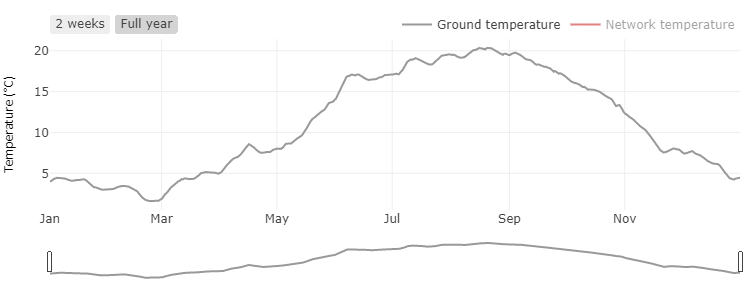The planning tool for district energy systems

# Ground temperature: Calculation and simulation of soil temperature profiles

Ground temperatures are of great importance for the calculation and simulation of 5GDHC networks, especially if uninsulated pipes are used. The temperatures of the soil affect the heat losses in district heating networks.

## What does the ground temperature depend on?

The decisive influence on the temperature changes in the ground is the weather. Air temperatures, solar radiation and precipitation are the decisive influencing variables. Essentially, the ground temperatures follow the air temperature. Basically, the profile of the soil temperature depends on the depth below the ground surface. Temperature layers deeper below the ground react less sensitively to temperature fluctuations of the air temperature. This is shown in Figure 1: Directly below the earth's surface, the earth's soil temperature directly follows the outside air temperature. At great depth, on the other hand, the ground temperature is constant. Interestingly, due to the time-delayed temperature propagation into the ground, the highest temperature during the year shifts in time with increasing depth. Thus, directly below the earth's surface, the temperature maximum is reached on the hottest summer day. The temperature maximum at a depth of a few meters, on the other hand, is not reached until autumn or even winter.Figure 1: Course of the ground temperature over the depth for different seasons

## How are temperature profiles calculated?

A variety of different calculation models have been developed for calculating ground temperatures based on weather data. The simplest models assume a sinusoidal curve and parameterize it with the help of a few weather data. These models are of limited use for estimating the earth's surface temperature. More detailed models are based on energy balances around layers of the earth. The most elaborate modeling approaches are based on finite element approaches. In these approaches, the soil is discretized into computational cells down to a certain depth, and an energy balance is established for each cell at each time step. The disadvantage of these dynamic FEM models is the high demand for input data and the high computational effort.
In the nPro tool, a medium accuracy calculation approach is chosen. This uses weather time series with hourly resolution (air temperature, etc.). The chosen approach provides sufficiently accurate temperature profiles for design calculations and allows a sufficiently accurate estimation of heat gains and losses in 5GDHC networks.Figure 2: Ground temperature over the course of a year at a depth of 1 m (calculated with the nPro tool).
In the nPro tool, annual profiles for ground temperature are calculated and used to estimate heat inputs and losses for 5GDHC networks.

## This might also interest you

### nPro Webtool

Plan and optimize district energy systems with nPro